# QUANTITAIVE APTITUDE.pptx

17 de Mar de 2023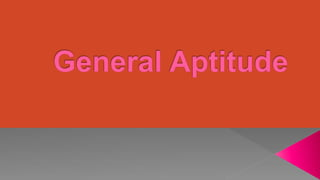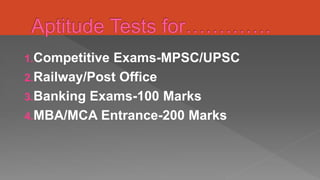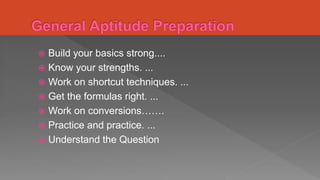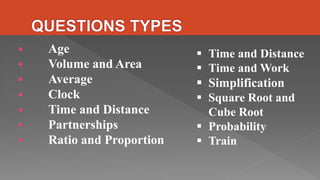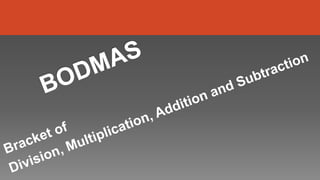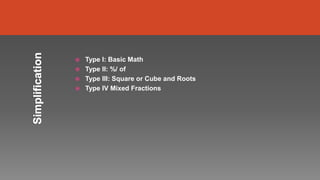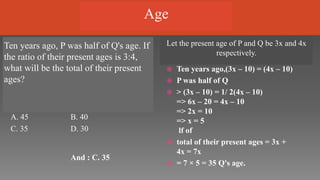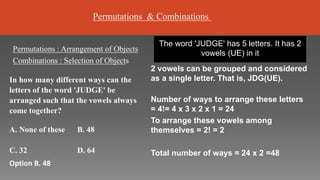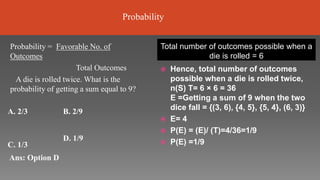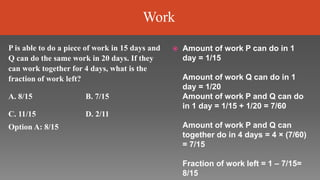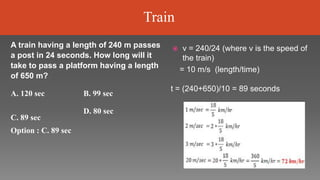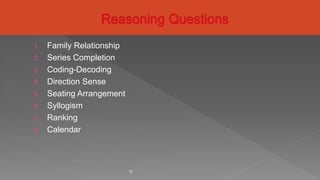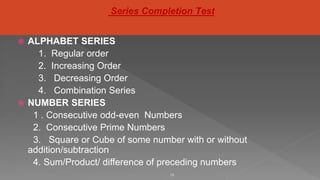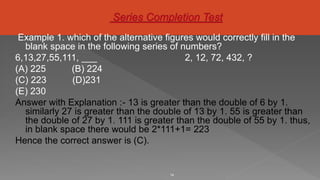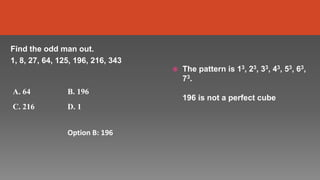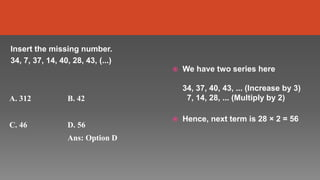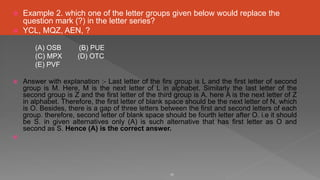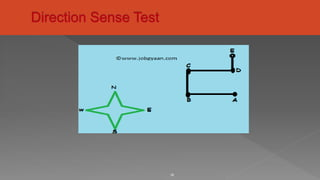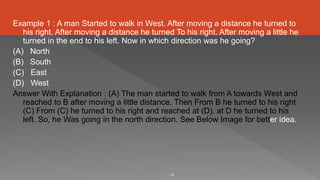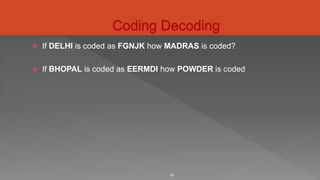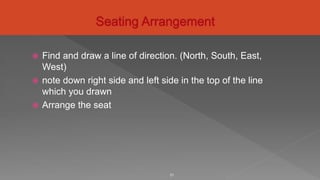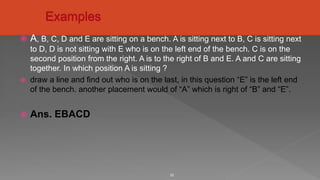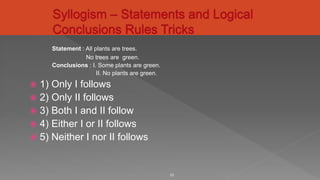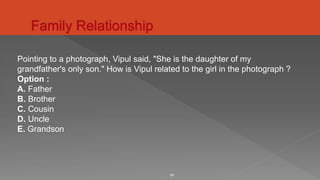1 de 24

### QUANTITAIVE APTITUDE.pptx

• 2. 1.Competitive Exams-MPSC/UPSC 2.Railway/Post Office 3.Banking Exams-100 Marks 4.MBA/MCA Entrance-200 Marks
• 3.  Build your basics strong....  Know your strengths. ...  Work on shortcut techniques. ...  Get the formulas right. ...  Work on conversions…….  Practice and practice. ...  Understand the Question
• 4.  Age  Volume and Area  Average  Clock  Time and Distance  Partnerships  Ratio and Proportion  Time and Distance  Time and Work  Simplification  Square Root and Cube Root  Probability  Train
• 6.  Type I: Basic Math  Type II: %/ of  Type III: Square or Cube and Roots  Type IV Mixed Fractions
• 7. Ten years ago, P was half of Q's age. If the ratio of their present ages is 3:4, what will be the total of their present ages? Let the present age of P and Q be 3x and 4x respectively. A. 45 B. 40 C. 35 D. 30 And : C. 35  Ten years ago,(3x – 10) = (4x – 10)  P was half of Q  > (3x – 10) = 1/ 2(4x – 10) => 6x – 20 = 4x – 10 => 2x = 10 => x = 5 lf of  total of their present ages = 3x + 4x = 7x  = 7 × 5 = 35 Q's age. Age
• 8. The word 'JUDGE' has 5 letters. It has 2 vowels (UE) in it 2 vowels can be grouped and considered as a single letter. That is, JDG(UE). Number of ways to arrange these letters = 4!= 4 x 3 x 2 x 1 = 24 To arrange these vowels among themselves = 2! = 2 Total number of ways = 24 x 2 =48 Permutations : Arrangement of Objects Combinations : Selection of Objects In how many different ways can the letters of the word 'JUDGE' be arranged such that the vowels always come together? A. None of these B. 48 C. 32 Option B. 48 D. 64 Permutations & Combinations
• 9. Total number of outcomes possible when a die is rolled = 6  Hence, total number of outcomes possible when a die is rolled twice, n(S) T= 6 × 6 = 36 E =Getting a sum of 9 when the two dice fall = {(3, 6), {4, 5}, {5, 4}, (6, 3)}  E= 4  P(E) = (E)/ (T)=4/36=1/9  P(E) =1/9 Probability = Favorable No. of Outcomes Total Outcomes A die is rolled twice. What is the probability of getting a sum equal to 9? A. 2/3 B. 2/9 C. 1/3 Ans: Option D D. 1/9 Probability
• 10.  Amount of work P can do in 1 day = 1/15 Amount of work Q can do in 1 day = 1/20 Amount of work P and Q can do in 1 day = 1/15 + 1/20 = 7/60 Amount of work P and Q can together do in 4 days = 4 × (7/60) = 7/15 Fraction of work left = 1 – 7/15= 8/15 P is able to do a piece of work in 15 days and Q can do the same work in 20 days. If they can work together for 4 days, what is the fraction of work left? A. 8/15 B. 7/15 C. 11/15 Option A: 8/15 D. 2/11 Work
• 11.  v = 240/24 (where v is the speed of the train) = 10 m/s (length/time) t = (240+650)/10 = 89 seconds A train having a length of 240 m passes a post in 24 seconds. How long will it take to pass a platform having a length of 650 m? A. 120 sec B. 99 sec C. 89 sec Option : C. 89 sec D. 80 sec Train
• 12. 1. Family Relationship 2. Series Completion 3. Coding-Decoding 4. Direction Sense 5. Seating Arrangement 6. Syllogism 7. Ranking 8. Calendar 12
• 13.  ALPHABET SERIES 1. Regular order 2. Increasing Order 3. Decreasing Order 4. Combination Series  NUMBER SERIES 1 . Consecutive odd-even Numbers 2. Consecutive Prime Numbers 3. Square or Cube of some number with or without addition/subtraction 4. Sum/Product/ difference of preceding numbers 13 Series Completion Test
• 14. Example 1. which of the alternative figures would correctly fill in the blank space in the following series of numbers? 6,13,27,55,111, ___ 2, 12, 72, 432, ? (A) 225 (B) 224 (C) 223 (D)231 (E) 230 Answer with Explanation :- 13 is greater than the double of 6 by 1. similarly 27 is greater than the double of 13 by 1. 55 is greater than the double of 27 by 1. 111 is greater than the double of 55 by 1. thus, in blank space there would be 2*111+1= 223 Hence the correct answer is (C). 14
• 15.  The pattern is 13, 23, 33, 43, 53, 63, 73. 196 is not a perfect cube Find the odd man out. 1, 8, 27, 64, 125, 196, 216, 343 A. 64 B. 196 C. 216 D. 1 Option B: 196
• 16.  We have two series here 34, 37, 40, 43, ... (Increase by 3) 7, 14, 28, ... (Multiply by 2)  Hence, next term is 28 × 2 = 56 Insert the missing number. 34, 7, 37, 14, 40, 28, 43, (...) A. 312 B. 42 C. 46 D. 56 Ans: Option D
• 17.  Example 2. which one of the letter groups given below would replace the question mark (?) in the letter series?  YCL, MQZ, AEN, ? (A) OSB (B) PUE (C) MPX (D) OTC (E) PVF  Answer with explanation :- Last letter of the firs group is L and the first letter of second group is M. Here, M is the next letter of L in alphabet. Similarly the last letter of the second group is Z and the first letter of the third group is A. here A is the next letter of Z in alphabet. Therefore, the first letter of blank space should be the next letter of N, which is O. Besides, there is a gap of three letters between the first and second letters of each group. therefore, second letter of blank space should be fourth letter after O. i.e it should be S. in given alternatives only (A) is such alternative that has first letter as O and second as S. Hence (A) is the correct answer.  17
• 18. 18
• 19. Example 1 : A man Started to walk in West. After moving a distance he turned to his right. After moving a distance he turned To his right. After moving a little he turned in the end to his left. Now in which direction was he going? (A) North (B) South (C) East (D) West Answer With Explanation : (A) The man started to walk from A towards West and reached to B after moving a little distance. Then From B he turned to his right (C) From (C) he turned to his right and reached at (D). at D he turned to his left. So, he Was going in the north direction. See Below Image for better idea. 19
• 20.  If DELHI is coded as FGNJK how MADRAS is coded?  If BHOPAL is coded as EERMDI how POWDER is coded 20
• 21.  Find and draw a line of direction. (North, South, East, West)  note down right side and left side in the top of the line which you drawn  Arrange the seat 21
• 22.  A, B, C, D and E are sitting on a bench. A is sitting next to B, C is sitting next to D, D is not sitting with E who is on the left end of the bench. C is on the second position from the right. A is to the right of B and E. A and C are sitting together. In which position A is sitting ?  draw a line and find out who is on the last, in this question “E” is the left end of the bench. another placement would of “A” which is right of “B” and “E”.  Ans. EBACD 22
• 23. Statement : All plants are trees. No trees are green. Conclusions : I. Some plants are green. II. No plants are green.  1) Only I follows  2) Only II follows  3) Both I and II follow  4) Either I or II follows  5) Neither I nor II follows 23
• 24. 24 Pointing to a photograph, Vipul said, "She is the daughter of my grandfather's only son." How is Vipul related to the girl in the photograph ? Option : A. Father B. Brother C. Cousin D. Uncle E. Grandson